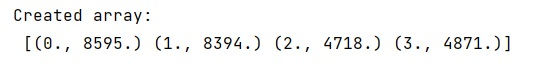# How to convert a dictionary to NumPy structured array?

Given a dictionary, we have to convert it into a NumPy structure array in Python.
Submitted by Pranit Sharma, on February 19, 2023

NumPy is an abbreviated form of Numerical Python. It is used for different types of scientific operations in python. Numpy is a vast library in python which is used for almost every kind of scientific or mathematical operation. It is itself an array which is a collection of various methods and functions for processing the arrays.

## Dictionary to NumPy structured array conversion

Suppose that we are given a dictionary that we need to convert to a NumPy structured array.

For this purpose, we can directly use the numpy array object to create a numpy array where we can pass the values by extracting them from dictionary using a comprehension statement but sometimes the user might face the following error in doing this:

To avoid this error, we will first convert the items of a dictionary into a list and then we will convert this list into numpy array using the array object. This will create the intermediate list of tuples and we will get a structured array of tuples as a result.

Let us understand with the help of an example,

## Python code to convert a dictionary to NumPy structured array

```# Import numpy
import numpy as np

# Creating a dict
d = {0:8595, 1:8394, 2: 4718, 3: 4871}

# Defining data type
names = ['id','data']
formats=['f8','f8']
dtype = dict( names = names, formats = formats)

# Creating an array
arr = np.array(list(d.items()),dtype = dtype)

# Display result
print("Created array:\n",arr)
```

Output: